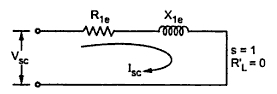### Blocked Rotor Tests

In this test, the rotor is locked and it is not allowed to rotate. Thus the slip s = 1 and RL' = R2' (1-s)/s is zero. If the motor is slip ring induction motor then the windings are short circuited at the slip rings.
The situation is exactly similar to the short circuit test on transformer. If under short circuit condition, if primary is excited with rated voltage, a large shot circuit current can flow which is dangerous from the windings point of view. So similar to the transformer short circuit test, the reduced voltage (about 10 to 15 % of rated voltage) just enough such that stator carries rated current is applied. Now the applied voltage Vsc, the input power Wsc and a short circuit current Isc are measured.
As RL' = 0, the equivalent circuit is exactly similar to that of a transformer and hence the calculations are similar to that of short circuit test on a transformer.
Vsc = Short circuit reduced voltage (line value)
Isc = Short circuit current (line value)
Wsc = Short circuit input power
Now       Wsc = √3Vsc Isc cosΦsc                       .............Line values

This gives us short circuit power factor of a motor.Fig. 1

Now the equivalent circuit is as shown in the Fig. 1.

where         Isc = Per phase value
This is equivalent resistance referred to stator.
Z1e = Vsc (per phase)/ Isc (per phase) = Equivalent impedance referred to stator.
During this test, the stator carries rated current hence the stator copper loss is also dominant. Similarly the rotor also carries short circuit current to produce dominant rotor copper loss. As the voltage is reduced, the iron loss which is proportional to voltage is negligibly small. The motor is at standstill hence mechanical loss i.e. friction and windage loss is absent. Hence we can write,
Wsc = Stator copper loss + Rotor copper loss
But it is necessary to obtain short circuit current when normal voltage is applied to the motor. This is practically not possible. But the reduced voltage test results can be used to find current ISN which is short circuit current if normal voltage is applied.
If             VL = Normal rated voltage (line value)
Vsc = Reduced short circuit voltage (line voltage)
where       Isc = Short circuit current at reduced voltage
Thus,        ISN = Short circuit current at normal voltage
Now power input is proportional to square of the current.
So   WSN = Short circuit input power at normal voltage
This cab be obtained as,

But at normal voltage core loss can not be negligible hence,
WSN = Core loss + Stator and rotor copper loss

Related articles :